# Alternating group

(diff) ← Older revision | Latest revision (diff) | Newer revision → (diff)
of degreeThe subgroup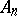of the symmetric group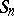consisting of all even permutations.is a normal subgroup inof index 2 and order. The permutations of, considered as permutations of the indices of variables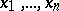, leave the alternating polynomial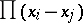invariant, hence the term "alternating group" . The group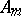may also be defined for infinite cardinal numbers, as the subgroup ofconsisting of all even permutations. If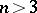, the groupis-fold transitive. For any, finite or infinite, except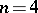, this group is simple; this fact plays an important role in the theory of solvability of algebraic equations by radicals.